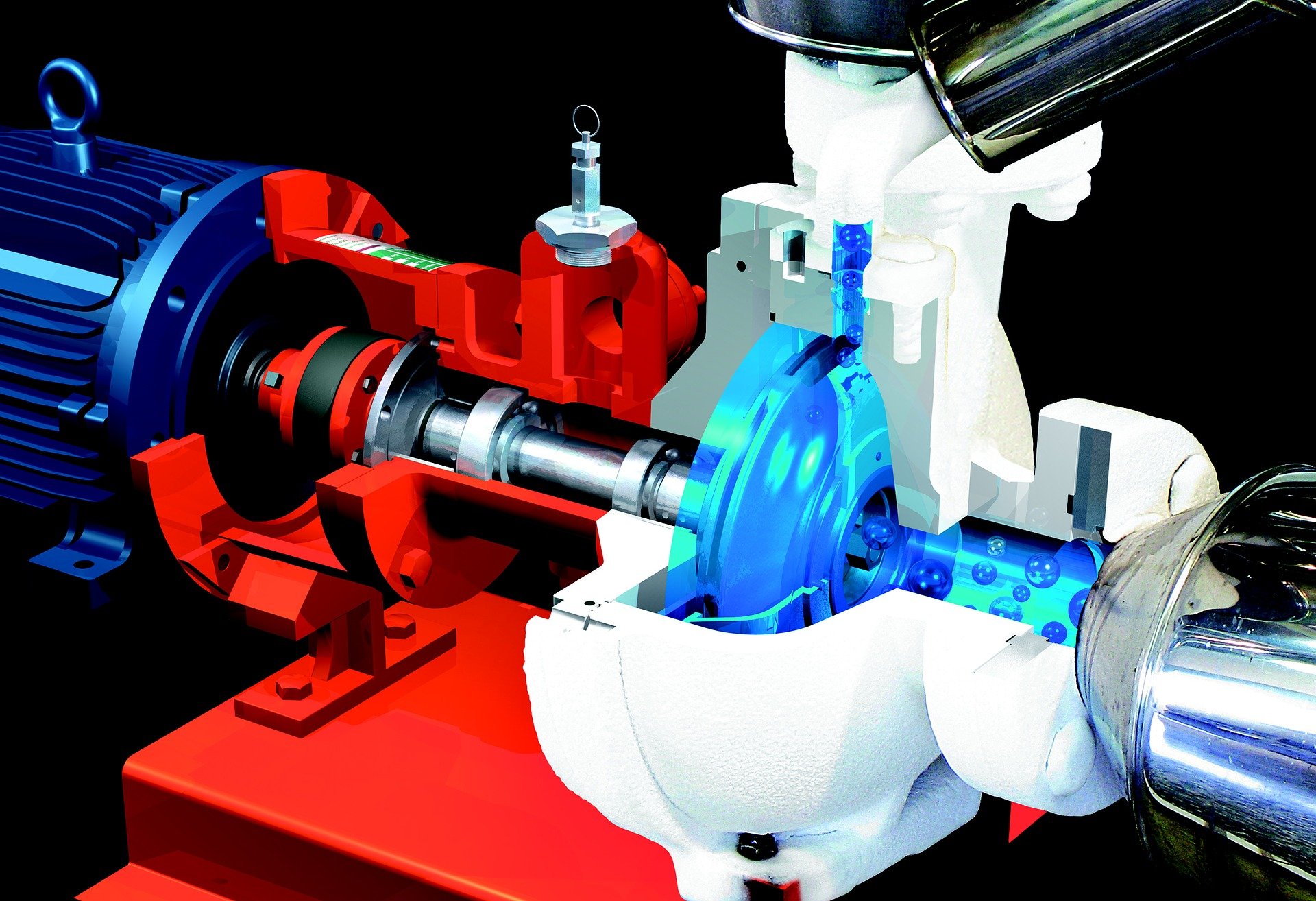# How to Calculate NSPH of Pump with Examples and Illustrations

NPSH of pump (net positive suction head) should be calculated/predicted in the beginning of pump sizing. The purpose is to check if the pump will work appropriately. Liquid pressure will lower right in inlet of pump impeller. If pressure reduction is lower than vapor pressure, liquid phase will change to vapor phase. The impeller rapidly collapses vapor bubbles which cause cavitation and damage.

In this post I will share you how to calculate NPSH available, which is net positive suction head calculated based on system arrangement. The value of NPSH available (NPSHA) shall be greater than NPSH required (NPSHR), which is a function of pump and to be specified by pump manufacturer.

NSPH of pump is calculated as follow:

NPSH available (ft, m) = absolute pressure (ft, m)vapor pressure (ft, m) –  line loss (ft, m) ± elevation difference (ft, m)

### EXAMPLE 1: Calculation of NPSH of Pump for Suction Lift

To calculate pressure in feet, use this equation:

h (ft) = 2.31 p/SG

where

p = pressure (psia)

SG = specific gravity

To calculate pressure in meter, use this equation:

h (ft) = 10.197 p/SG

where

p = pressure (bara)

SG = specific gravity

Figure below is the first example of piping arrangement to calculate NPSH of pump for suction lift.

For elevation difference, always use the lowest elevation difference (LLL, Liquid Level).

SG of water = 1

NPSH available (ft, m) = absolute pressure (ft, m)vapor pressure (ft, m) –  line loss (ft, m) ± elevation difference (ft, m)

NPSHA = 2.31 x 14.7/12.31 x 0.5/1 310 = 19.8 ft. (for suction lift, elevation difference is minus).

### EXAMPLE 2: Calculation of NPSH of Pump for Liquid at Boiling Point or Pressured Drum

Figure below illustrate piping configuration to calculate NPSH of pump for liquid at boiling point.